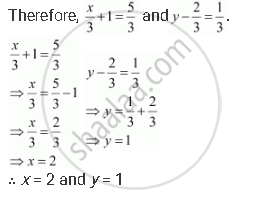# If (x3+1,y-23)=(53,13) find the values of x and y. - Mathematics

Sum

if (x/3 + 1, y - 2/3) = (5/3, 1/3) find the values of x and y.

#### Solution

It is given that(x/3 + 1, y - 2/3) = (5/3, 1/3).

Since the ordered pairs are equal, the corresponding elements will also be equal.Concept: Cartesian Product of Sets
Is there an error in this question or solution?

#### APPEARS IN

NCERT Class 11 Mathematics Textbook
Chapter 2 Relations and Functions
Q 2.1 | Page 33